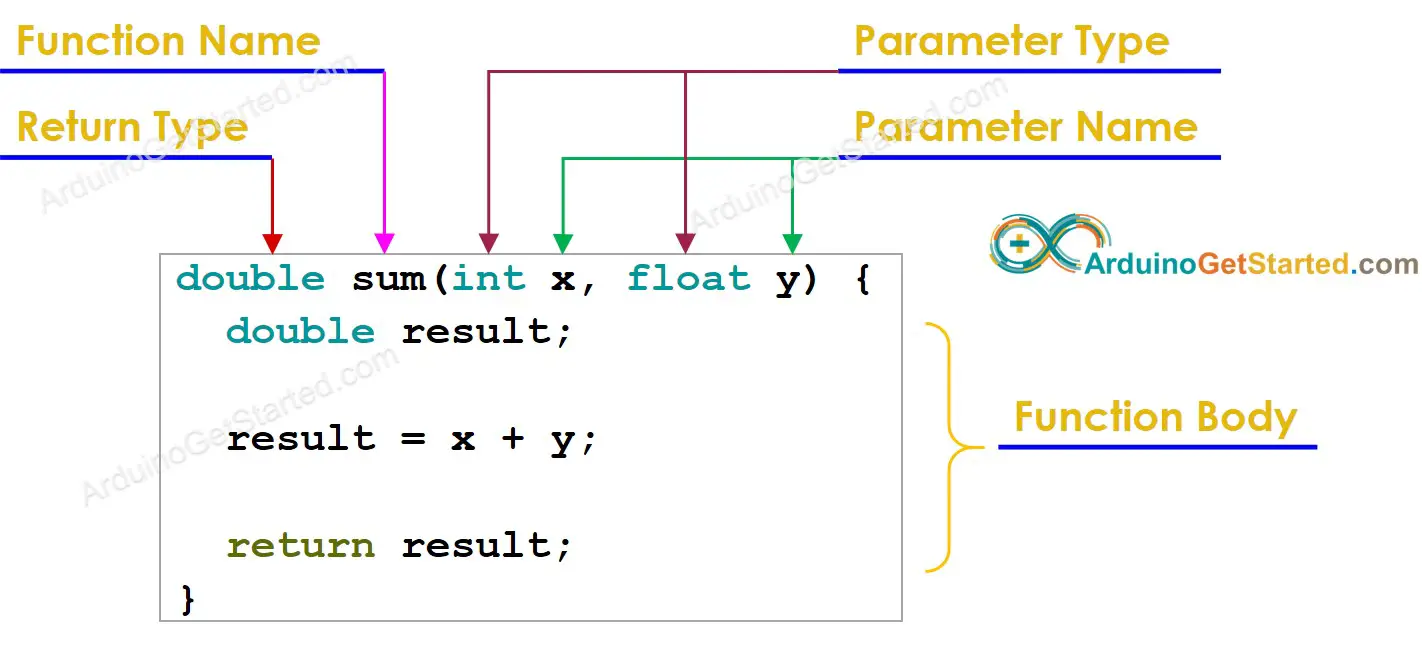# Function

## What is Function in Arduino

A function is pieces of code that perform a specific task and may return to a value. Instead of repeating the same pieces of code in multple places, The function group it into a single place and then call it at where it needed.

## Why Use Function

• Function makes the whole sketch smaller and more compact.
• Function makes it easier to reuse code in other programs by making it more modular
• Function increases the readability of the code.
• Function conceives and organizes the program.
• Function reduces the chances of errors.
• Function makes easier do debug program.
• Function avoids the repetition of the set of statements or codes.
• Function allows us to divide a complex code or program into a simpler one.

There are two required functions in an Arduino sketch: setup() and loop(). Other functions must be created outside the brackets of those two functions.

## How to use Function

To use a function, we need to do two works:

• Declaring a function
• Calling a function

### Declaring a function

To create a function, we need to know the structure of a function.

A function includes four parts:

• Return Type: (mandatory)
• Function Name: (mandatory)
• Parameters: (optional) includes parameter type and paremeter name
• Function Body: (mandatory)

The syntax:

returnType functionName(parameterType1 parameter1, parameterType2 parameter2 ...) { // function body here }

For example:#### Return type

The function do some specific works. It may return a value as the result of the works. We MUST specify which type of value such as boolean, byte, char, double, float, int, long, String() ...

If there is no value to returm, the returm type is void

#### Function Name

The function name consists of a name specified to the function. It represents the real body of the function. It is used to call function.

#### Parameters

The parameters are optional. it can be nothing, one or more parameters. Each parameter includes the data type of parameter and parameter name. The parameters are data to pass to function when it is called. The data type of parameters can be different

In other works, paremeters are input and the return value is output.

#### Function Body

The function body is the implementation of the function. It is placed in side a {} (curly braces).

If the return type is void, it does not need to use return to terminate the function. Otherwise, The return MUST be used.

### Calling a Function

After declaring a function, calling the function is simple. It just need to put function name, parameters and terminated by a comma. In case of the return type is not void, we may need to use a variable to store the return value.

The syntax:

functionName(parameter1, parameter2 ...); variable = functionName(parameter1, parameter2 ...);

In the function call. we do not put the return type and parameter type.

For example.

double variable; variable = sum(3, 5);

## Example

void setup() { Serial.begin(9600); int x = 2; float y = 3.5; double z ; z = sum(x, y); Serial.println(z); z = sum(x, 10.1); Serial.println(z); z = sum(5, 10.1); Serial.println(z); } void loop() { } double sum(int x, float y) { double result; result = x + y; return result; }

The output on Serial Monitor:

COM6
5.50 12.10 15.10
Autoscroll Show timestamp
Clear output
9600 baud
Newline

※ NOTES AND WARNINGS:

Why are the [setup()](BASE_URL/reference/arduino-setup) and [loop()](BASE_URL/reference/arduino-loop) functions declared without being called ?

⇒ The setup() and loop() functions actually are called by Arduino main program. In other words, these functions are called in background, we do not need to care about it.